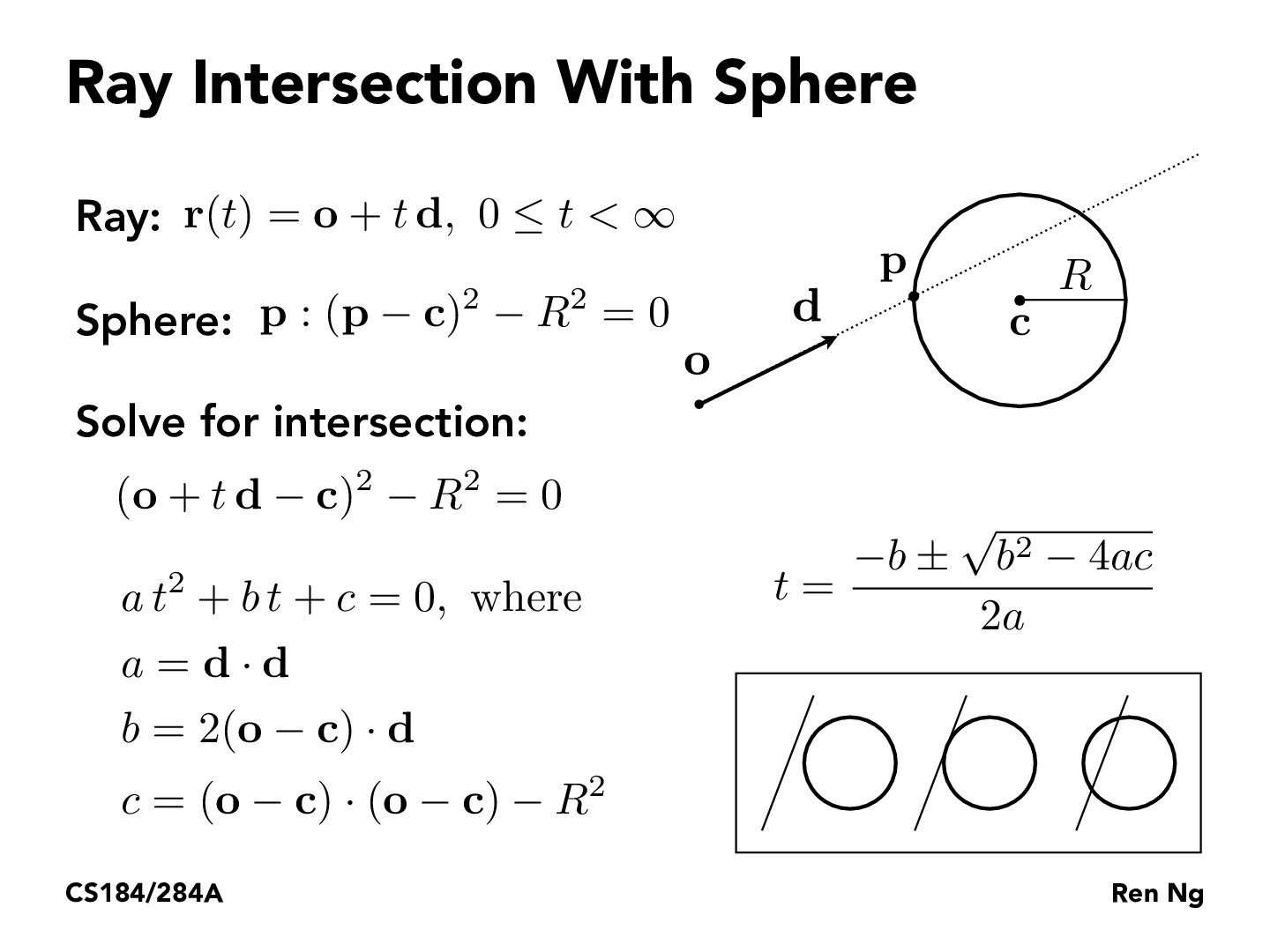Lecture 10: Ray Tracing (21)rileylyman184

This instantly made me think of the work we have been doing with Splines and Bezier curves. If, in 2 dimensions, you wanted to see if the ray intersected a Bezier curve, then we could simply use this method with the polynomial description of the curve to test for intersection points. I guess in this case, it would be better to explicitly solve for the close-form of the Bezier curve rather than use de Casteljau's algorithm, since we want to be able to do the algebraic manipulations required to test if the ray intersects the curve.

Just like this case with the circle, the maximum number of intersections with the Bezier curve would be the degree of the curve. We would just need to make sure that the intersections lie in the range t=0..1, since that is where the curve is defined. However, now there are two t variables, one for the ray and the other for the Bezier curve. We will need to do some bookkeeping to make sure these do not conflict.frgalvan

Did I hear this correctly, on the lecture video, that the general concept here is treating this circle like a triangle mesh for determining intersections with Ray Tracing?jinwoopark1673

Is the formula here also applicable to 3D space? I feel like any ray that penetrates the sphere with some angle would be analogous to a circle with smaller radius.hfan9

@frgalvan Professor said that sometimes, you can algebraically solve for the intersction, and othertimes you can't. When you can algebraically solve (e.g. for triangles/planes, and this sphere here), it gives a closed form solution. When you can't, you can approximate the surface you're intersecting with a triangle mesh, then test with the triangles in the mesh. The sphere is not complicated and the math works out somewhat nicely, so for this sphere approximating with a mesh is not necessary.

@jinwoopark1673 The formula here is for 3D space. Note that the sphere equation uses vectors $\mathbf{p}$ and $\mathbf{c}$, which generalize to arbitrary dimensions. The square on the vectors should mean dot product with itself (to find the distance). When you simplify to the formula at the end, the dot products produce scalars, which you can just plug in. In general though, I think it'd hard to approximate the sphere with a circle, since you'd have to find the intersection with the sphere first to get a point, and then there's infinite circles on the sphere's surface that go through that point anyways.bufudash

I didn't get this from the lecture. How can we treat Ray Intersection with Sphere which is a 3D action as a 2D model as shown in slides?aninrusimha

@bufudash I believe a sphere in this case means in a sphere in any dimension. Notice how all of the bold letters are vectors, and the dimension of the vectors is not specified. Regardless of what dimension we are in, all equations are still true

You must be enrolled in the course to comment